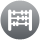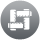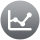## Mathematics

In mathematics, students are encouraged to become critical thinkers, who have a wide range of mathematical tools at their disposal for use in investigations, applications and analysis. Students engage in a curriculum that is motivating and intellectually rigorous.Mathematics provides a framework for logical thinking deductive reasoning as well as being a means of symbolic communication that is powerful, concise, logical and unambiguous. It is a means by which people can manage and understand their environment.

Through study in this area, students will develop the essential mathematical skills of abstraction, proof, investigation, modelling and problem solving.

Students studying Mathematics at Dalyellup College are encouraged to explore and appreciate the power of mathematical reasoning. Mathematics provides students with essential mathematical skills and knowledge in Number and Algebra, Measurement and Geometry, and Statistics and Probability. It develops the numeracy capabilities that all students need in their personal, work and civic life, and provides the fundamentals on which mathematical specialties and professional applications of mathematics are built.

##Number and AlgebraDevelopment of problem solving and reasoning

Students apply number sense and strategies for counting and representing numbers. They explore the magnitude and properties of numbers. They apply a range of strategies for computation and understand the connections between operations. They recognise patterns and understand the concepts of variable and function. They build on their understanding of the number system to describe relationships and formulate generalisations. They recognise equivalence and solve equations and inequalities. They apply their number and algebra skills to conduct investigations, solve problems and communicate their reasoning.

##Measurement and GeometryDevelopment of skills in geometry and understanding of units of measurement

Students develop an increasingly sophisticated understanding of size, shape, relative position and movement of two-dimensional figures in the plane and three-dimensional objects in space. They investigate properties and apply their understanding of them to define, compare and construct figures and objects. They learn to develop geometric arguments. They make meaningful measurements of quantities, choosing appropriate metric units of measurement. They build an understanding of the connections between units and calculate derived measures such as area, speed and density.

##Statistics and ProbabilityDevelopment of statistical and data evaluation skills

Students recognise and analyse data and draw inferences. They represent, summarise and interpret data and undertake purposeful investigations involving the collection and interpretation of data. They assess likelihood and assign probabilities using experimental and theoretical approaches. They develop an increasingly sophisticated ability to critically evaluate chance and data concepts and make reasoned judgments and decisions, as well as building skills to critically evaluate statistical information and develop intuitions about data.

### Year 11 and 12 Mathematics Course

The Mathematics courses available to senior students allows for multiple entry points to accommodate the diversity of students’ mathematics development at the point of entry into senior school as well as the diversity of post school destinations.

Students can choose units based on their particular need: to develop their general mathematical skills for further training or employment, to enable university entry where further mathematics may not be essential, to prepare them for university courses where further mathematics studies is required or for preparation for higher level training in technical areas.